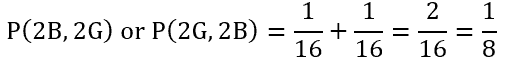# PCAT Quantitative Reasoning – Offspring Probability

If a couple has 4 children, what is the probability that the last two children are the opposite sex of the first two children?##### Explanation

A is correct. There are two different outcomes that would result in the last two children having the opposite sex of the first two children. Keep in mind that this assumes that the first two children have the same sex and the last two children have the same sex:The probability of having two boys and then two girls is equal to the product of the probabilities for having a child of each sex (i.e., probability of having a boy for the first child (1/2) times the probability of having a boy as the second child (1/2) and so on):The same equation applies for the second outcome, having two girls and then two boys:To find the total probability for either one or the other event occur, we must consider this an “or” problem and add these two probability values:#### Submit a Comment

This site uses Akismet to reduce spam. Learn how your comment data is processed.